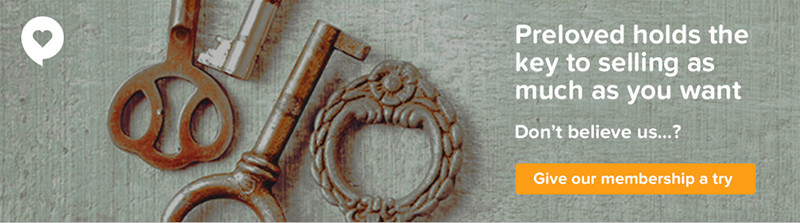I AM ACCEPTING OFFERS FOR all THESE MAGS 4 BIG BOXES FULL... BEST PRICE PLEASE - no splitting

Brit’ Mag
=
2008
=
3
En France
=
2007
=
1
EVERYTHING FRANCE = 2004 =
3
French Entrée
=
2013
=
1
FRANCE Magazine
= 2003 = 2
2004 = 9
2005 = 2
2007 = 1
2009 = 1
2014 = 1
2016 = 1
2017 = 1
French Magazine
=
2002
=
2
2003 = 1 2004 = 4 2005
= 1 2007
=
1
=
9
FRENCH Property News
= 2001
= 1 THE PAPER ONE
2001
MAGAZINE = 10
2002 = 10
2003 = 9
2004 = 11
2005 = 12
2006 = 10
2007 = 10
2008 = 7
2009 = 12
2010 = 12
2011 = 12
2012 = 12
2013 = 10
2014 = 12
2015 = 11
2016 = 5
2017 = 1
=
168
Living France
= 2000 = 2
2001 = 11
2002 = 3
2003 = 5
2004
= 10
2005 = 6
2008 = 3
2009 = 1
2012 = 1
2014 = 2
= 44
(Place in the Sun ) France = 2006
=
1
The Winter Traveller in France = 2004-2005
=
1
3 + 1 + 3 + 1 + 18 + 9 = 35
FPN = 1+10+10+9+11+12+10+10+7+12+12+12+12+10+12+11+5+1= 167
LF = 44
1+1 = 2
TOTAL
=
248 =
PRICE : could be £100 or slightly lower if ALL BOUGHT
THEY ARE SELLING FROM £1 TO £3.75 EACH = e BAY
I have read all of these and they are in excellent condition - one has no cover, all the rest are in lovely condition - no cut outs or rips etc.
12 FOR £5 OR £1 for 2
OR Very NEAR OFFER FOR ALL
POSTAGE + PACKING EXTRA
PICK UP FREE
NEAR M56 J10
WA4 5PT